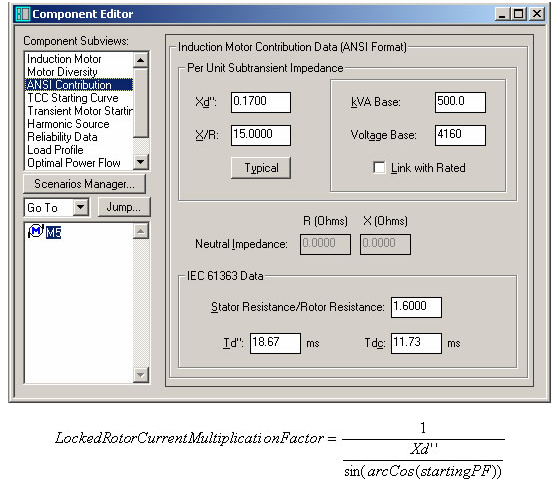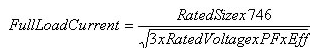• Sales
• Library Model Request
• Technical Support

# How is the induction motor startup current multiplication factor calculated in a load flow study?

Solution:
The startup current multiplication factor is calculated from the inverse motor subtransient impedance. This can be found in the Component Editor under the ANSI/IEC Contribution subview window along with the starting power:This Locked Rotor Current Multiplication Factor is multiplied by the Full Load Nominal Current of the motor. The Full Load Nominal Current is calculated from the motor rated values defined in the Induction motor sub-view window.